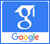# Natural convection## Heat transfer investigation of Non-Newtonian Fluid between two vertically infinite flat plates by numerical and analytical solutions

Published on: 18th May, 2017

OCLC Number/Unique Identifier: 7317592196

In natural convection, the fluid motion occurs by natural means such as buoyancy. Heat transfer by natural convection happens in many physical problems and engineering applications such as geothermal systems, heat exchangers, petroleum reservoirs and nuclear waste repositories. These problems and phenomena are modeled by ordinary or partial differential equations. In most cases, experimental solutions cannot be applied to these problems, so these equations should be solved using special techniques. In this paper, natural convection of a non-Newtonian fluid flow between two vertical flat plates is investigated analytically and numerically. Collocation Method (CM) and fourth-order Runge -Kutta numerical method (NUM) are used to solve the present problem. These methods are powerful and convenient algorithms in finding the solutions for the equations. While these are capable of reducing the size of calculations. In order to compare with exact solution, velocity and temperature profiles are shown graphically. The obtained results are valid with significant accuracy.